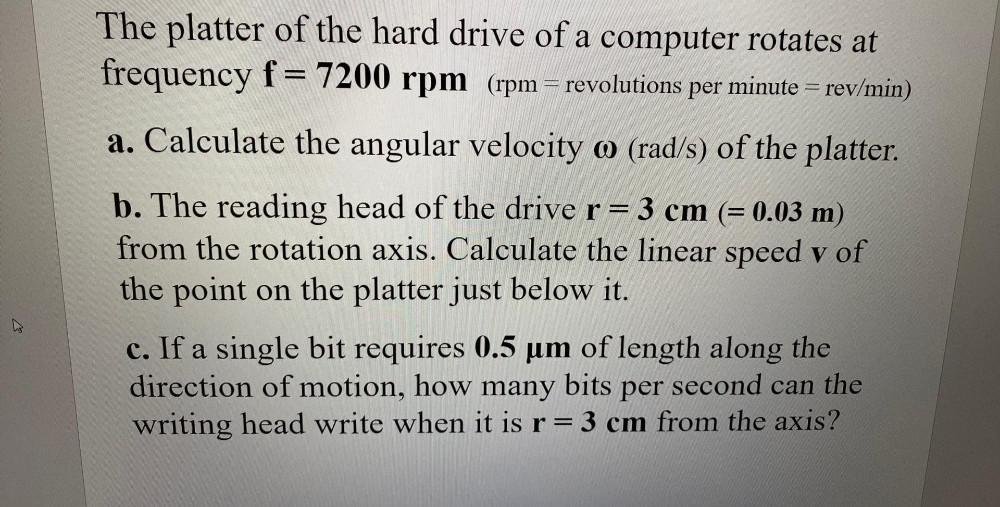Question:

# The platter of the hard drive of a computer rotates at frequency f= 7200 rpm (rpm = revolutions per minute = rev/min) a. CalculaThe platter of the hard drive of a computer rotates at frequency f= 7200 rpm (rpm = revolutions per minute = rev/min) a. Calculate the angular velocity o (rad/s) of the platter. b. The reading head of the drive r= 3 cm (= 0.03 m) from the rotation axis. Calculate the linear speed v of the point on the platter just below it. c. If a single bit requires 0.5 um of length along the direction of motion, how many bits per second can the writing head write when it is r= 3 cm from the axis? a